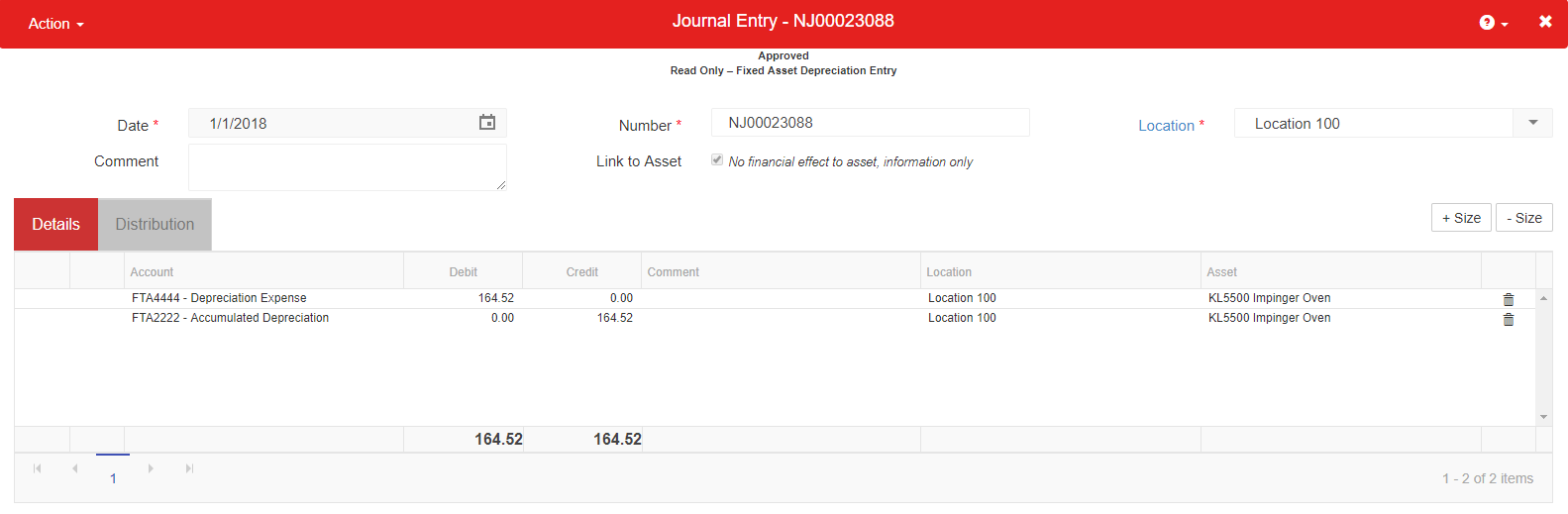Through the Asset Class or Asset record, the Deprecation Method is assigned to the Asset. Important aspects to note include the following:

• Each Depreciation record will be created with a status of 'Unposted' once the Asset has been 'Placed in Service'
• One Depreciation record will be created for each Period and each Book for the Effective Life of the Asset
• For example, if an Asset had 13 periods of Depreciation and was utilizing three Active Books, a total of 39 Depreciation records would be created (3 * 13)
• Each Depreciation record will Debit the 'Depreciation Expense' Account for the Asset and Credit the 'Accumulated Depreciation' Account
• These Accounts are set on the Asset Class or assigned on the 'General tab' of the Asset record.Depreciation Methods:

• Straight Line - Asset Depreciation that results in the same equal amount of depreciation expense for each Period over the Effective Life of the Asset (with a possible amount variation in the final posting)
• None - No Depreciation records will be created, Asset will have no financial value